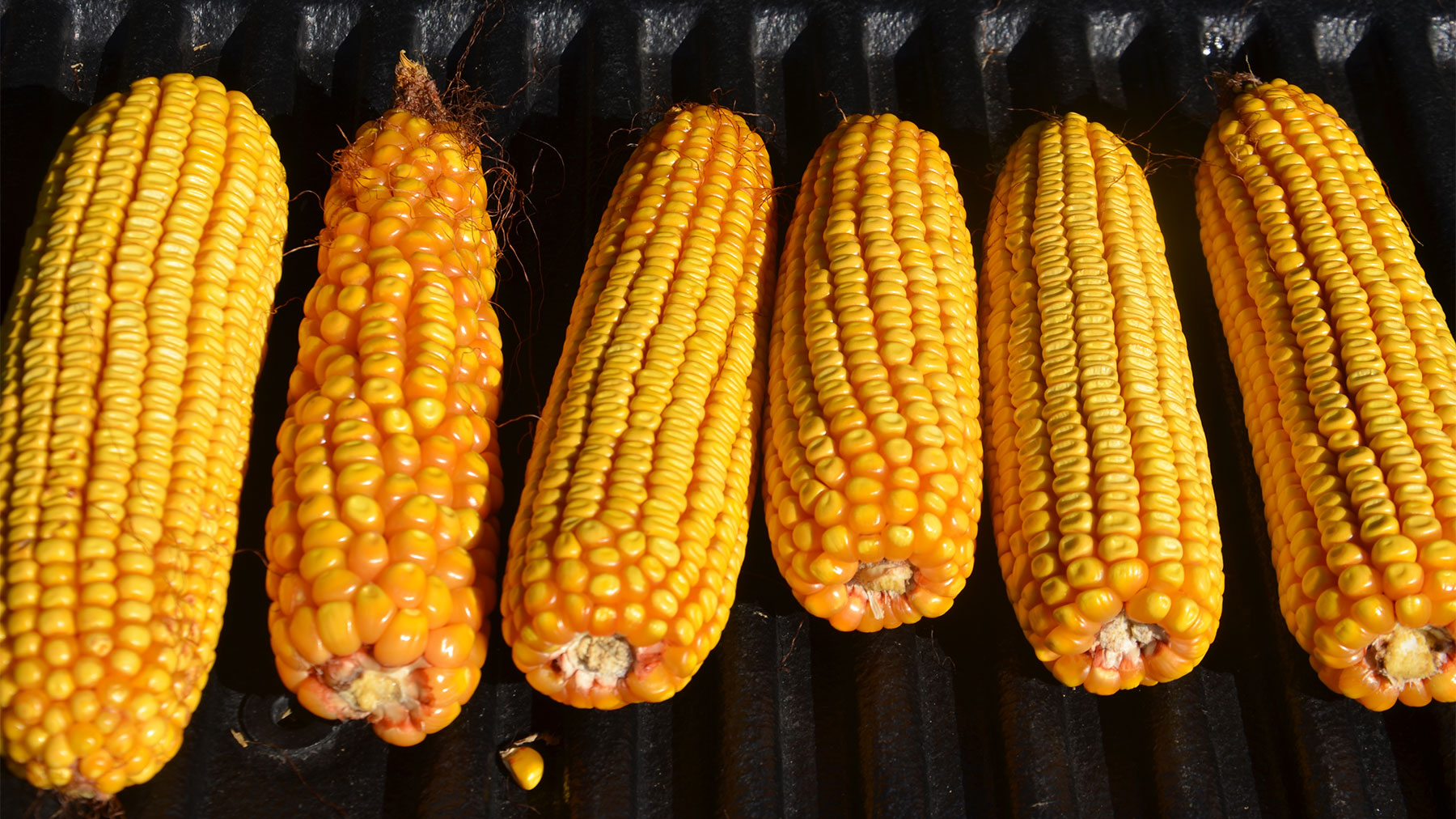# Yield estimate formula: Small changes have big effectYield estimate formula: Small changes have big effect

Corn Illustrated: Longer ear, girthier ear, deeper kernels — these variables can change yields quickly.RANDOM SAMPLE: Which of these ears you find when you pull ears at random for yield estimates will impact your final yield projections. Tom J. Bechman

Fall is the favorite time of year for some agronomists. It’s time to estimate corn yields and see how close they can come.

“They can get fairly close if they sample enough areas at random,” says Dan Quinn, Purdue Extension corn specialist. “What anyone who estimates learns quickly, however, is that small changes in any one of the factors that impact yield can move the needle on yields significantly.”

Here is the formula Quinn relies on for estimating corn yields: It’s number of ears per 1/1,000 acre times number of rows around the ear, times number of kernels per row, divided by a fudge factor based on the number of kernels in a bushel volume.

To make estimating simple, use a rope marked at 17 feet, 5 inches for 30-inch corn rows, Quinn advises. That’s 1/1,000 acre. Pick the spot at random and repeat the estimate at several locations in the field. Average the results to get a reasonable whole-field estimate.

Pull three ears to evaluate the number of rows and number of kernels per row at each location. Be sure to pull ears at random instead of looking for the biggest ears, Quinn advises. Next, select the fudge factor — often ranging from 90 to 70, depending upon depth of kernels — with 90 being shallow kernels, 70 being very deep kernels, and 80 an average number for modern hybrids.

## Yield estimate examples

Here are five examples of how one variable can affect total yield. Number for rows of kernels around are an average of number of rows found on three ears selected at random.

1. Number of ears per acre. Suppose you find 30 ears per acre, with 16.7 kernels around and 38 kernels per row. The fudge factor is 80. So, the formula is 30 x 16.7 x 38 / 80 = 238 bushels per acre.

Now, suppose you only count 28 ears per 1/1,000 acre. The equation is 28 x 16.7 x 38 / 80 = 222.1, almost 16 bushels less.

2. Rows of kernels per ear. Now you find ears with 18 kernels around, but still with 38 kernels per row and the fudge factor at 80. The math is 30 x 18 x 38 / by 80 = 256.5 bushels per acre. That’s up 18.5 bushels from the first example, based on another 1.3 rows around per ear.

3. Kernels per row. You have another 30 ears per 1/1,000 acre, with 16.7 rows per ear and 36 kernels per row. The formula is 30 x 16.7 x 36 / 80 = 225.5 bushels per acre, down 12.5 bushels per acre from the original example — all because you gave up two kernels per row per ear.

4. Fudge factor due to poor weather conditions. Take the original 30 ears per 1/1,000 acre, with 16.7 rows around and 38 kernels per row. Inconsistent weather hurt grain fill and the kernels are smaller, so the equation is 30 x 16.7 x 38 / 90 = 211.5 bushels per acre. That’s off 26.5 bushels from the original 238-bushel yield, simply due to less-than-average grain fill.

5. Fudge factor due to plenty of rain. Same 30 ears, but kernels are plump, so you use 75 as the fudge factor. Math is 30 x 16.7 x 38 / 75 = 253.9 bushels per acre, up 15.9 bushels from the original 238-bushel yield.